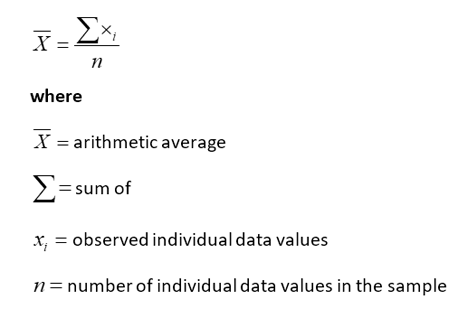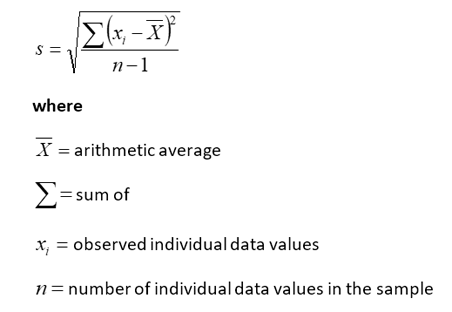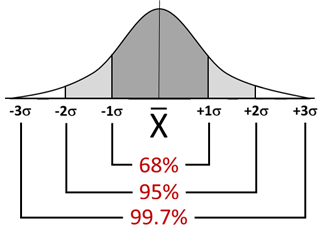### Statistical Process Control 101

Learn all about SPC for manufacturing.

# Distributions

To begin evaluating the type of variation in a process, one must evaluate distributions of data—as Deming plotted the drop results in his Funnel Experiment. The best way to visualize the distribution of results coming from a process is through histograms. A histogram is frequency distribution that graphically shows the number of times each given measured value occurs. These histograms show basic process output information, such as the central location, the width and the shape(s) of the data spread.

## Location: Measure of Central Tendency

There are three measures of histogram’s central location, or tendency:

• Mean (the arithmetic average)
• Median (the midpoint)
• Mode (the most frequent)

When compared, these measures show how data are grouped around a center, thus describing the central tendency of the data. When a distribution is exactly symmetrical, the mean, mode and median are equal.

### Formula for estimating population mean

To estimate a population mean, use the following equation:## Dispersion: Spread of the Data

The two basic measures of spread are the range (the difference between the highest value and the lowest value in the sample) and the standard deviation (the average absolute distance each individual value falls from the distribution’s mean). A large range or a high standard deviation indicate more dispersion, or variation of values within the sample set.

### Formula for estimating standard deviation

To estimate the standard deviation of a population, use the following equation:Some other measures of the shape of a distribution are skewness, which describes the symmetry of the distribution, and kurtosis, which describes the peak of the distribution.

## Central Limit Theorem

A group of sample averages tends to be normally distributed. A normal distribution exhibits the following characteristics:

• The shape is symmetrical about the mean.
• The mean, mode, and median are essentially equal.
• The curve is bell shaped.
• Values are concentrated around the mean.
• The total area under the curve equals 100%, with 50% of the data to the left side of the mean and 50% to the right.
• 99.73% of the values fall within ±3 standard deviations of the mean.

As the sample size increases, the tendency toward normality improves. This enables users to form conclusions about populations based on sample statistics.### Take the first step from quality to excellence

Take the Next Steps